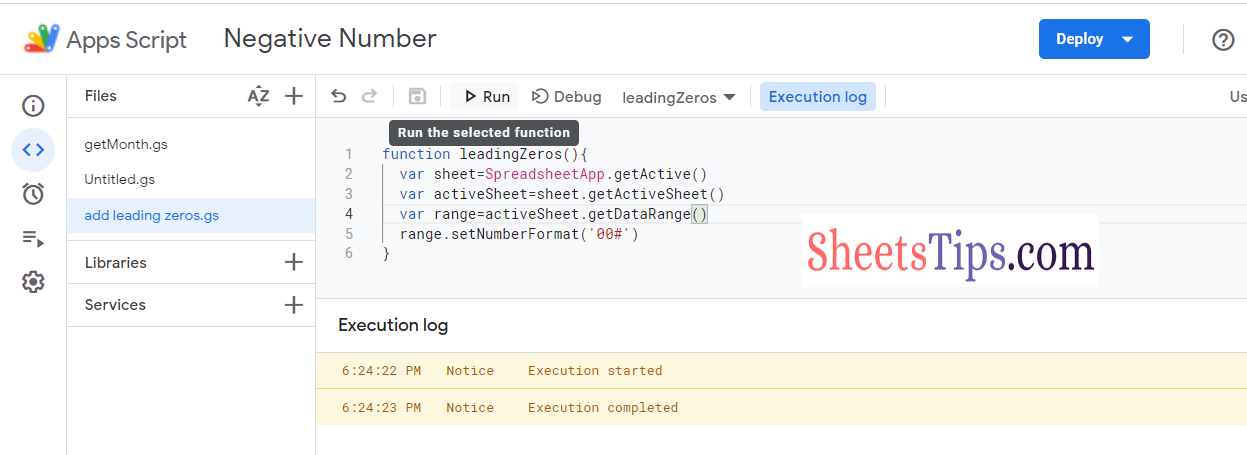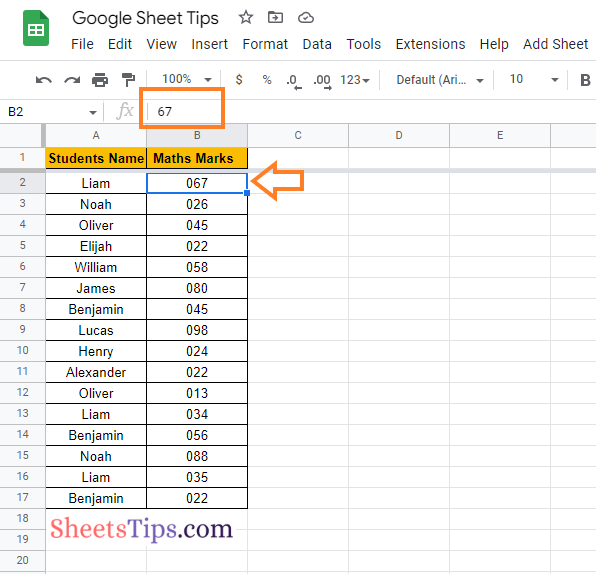One of the easiest methods to keep leading zeros in Google Sheets is to use the Custom Number format feature. The detailed steps on how to get this done in Google Sheets are given below:

• 2nd Step: On the homepage, click on the Format tab and choose “Number” from the drop down menu.
• 3rd Step: Choose “Custom Number Format” from the drop down box.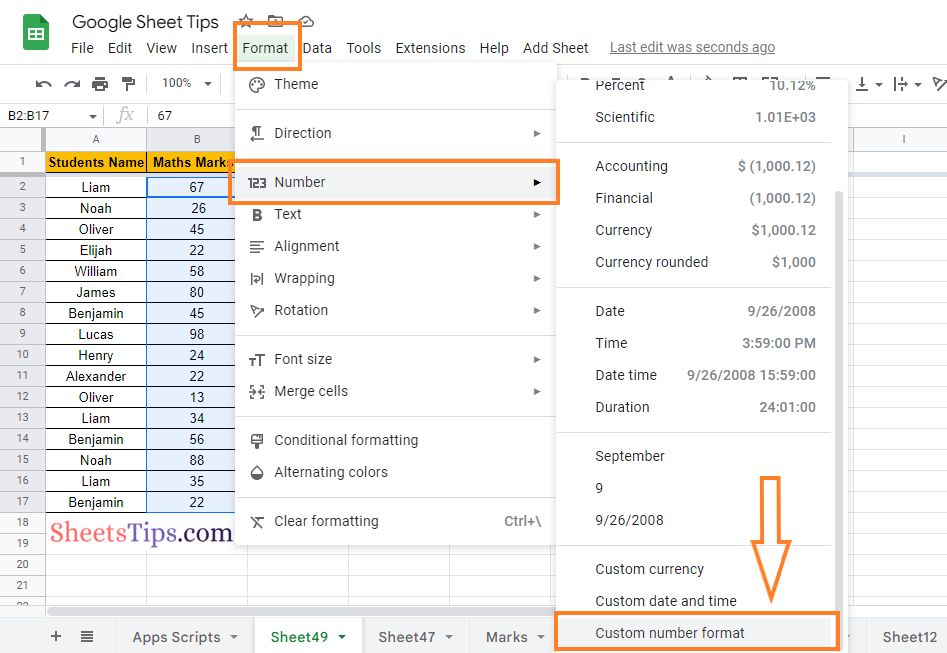• 4th Step: In the custom number format, enter the number as “000“. Here I have added only 3 zeros since I need to add only one zero before the number. Depending on the number of leading zeros you need to add, you can calculate this formula.
• 5th Step: Press the “Apply” button and you will find the results as shown in the image given below.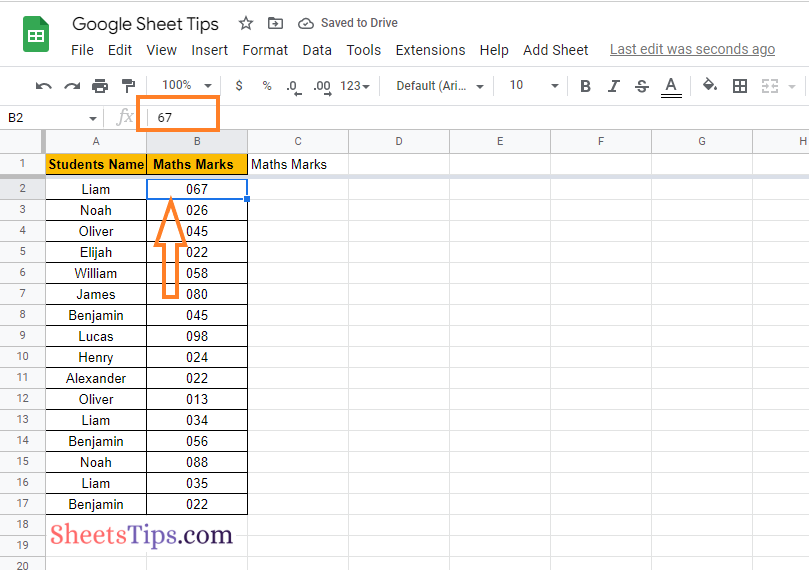• 2nd Step: On the homepage, click on the cell where you want to add the leading zeros.
• 3rd Step: Enter the symbol ‘(apostrophe) and enter the number of zeros you want as shown here.
• 4th Step: Press the “Return” key and you will find the results as shown in the image given below.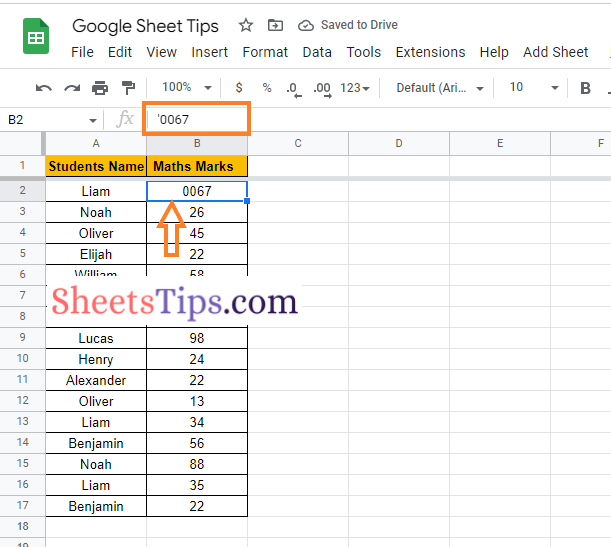The TEXT function is another simple way to add leading zeros in a Google Spreadsheet. The detailed steps on how to use the TEXT function to add zeros in Google Sheets are given below:

• 2nd Step: Now on the homepage, move to the cell where you would like to add zeros.
• 3rd Step: Enter the formula “=TEXT (B2,”000″)“.
• 4th Step: Press the “Return” key and you will find the results shown in the image given below.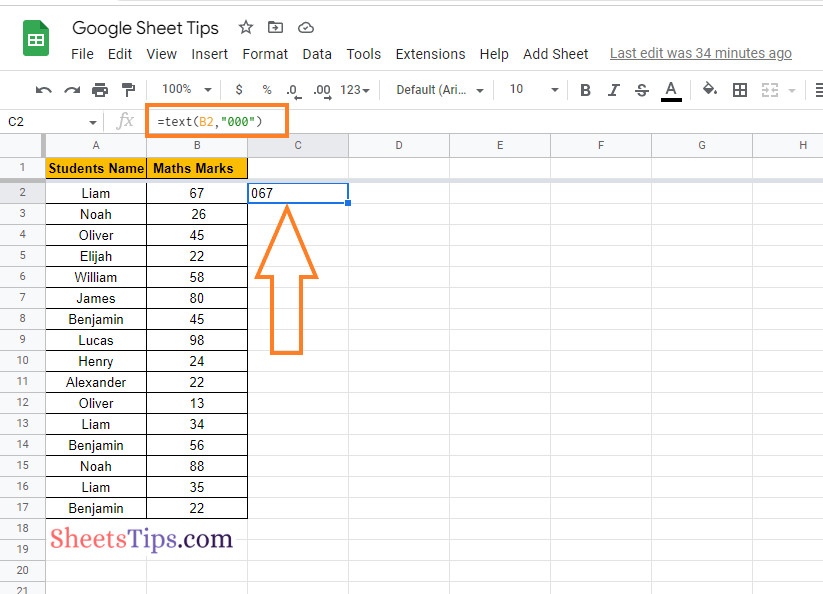Apart from the TEXT function, we can also use the RIGHT function in Google Sheets, which helps us to add leading zeros. The detailed steps on how to add leading zeros in a spreadsheet using the RIGHT function are outlined below:

• 2nd Step: On the homepage, move to the cell where you want to use the RIGHT function.
• 3rd Step: Enter the formula “=RIGHT(“000” & B2,3)“.
• 4th Step: Press the “Return” key and you will find the results as shown in the image given below.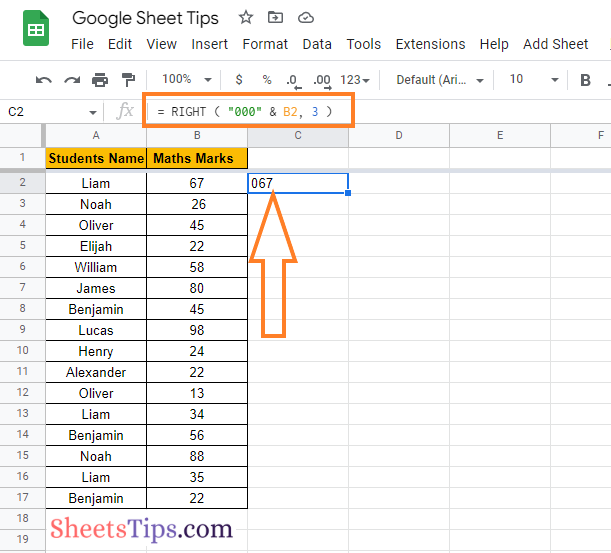Most spreadsheet users would be familiar with the CONCATENATE function. If you are also one of them, then adding a leading zero is much easier in Google Sheets using the CONCATENATE function. The detailed steps on how to do this in Google Sheets are given below:

• 2nd Step: On the homepage, move to the cell where you want to use the CONCATENATE function.
• 3rd Step: Enter the formula “=CONCATENATE (“0,” “B2”)“.
• 4th Step: Press the “Enter” key and you will find the results as shown in the image given below.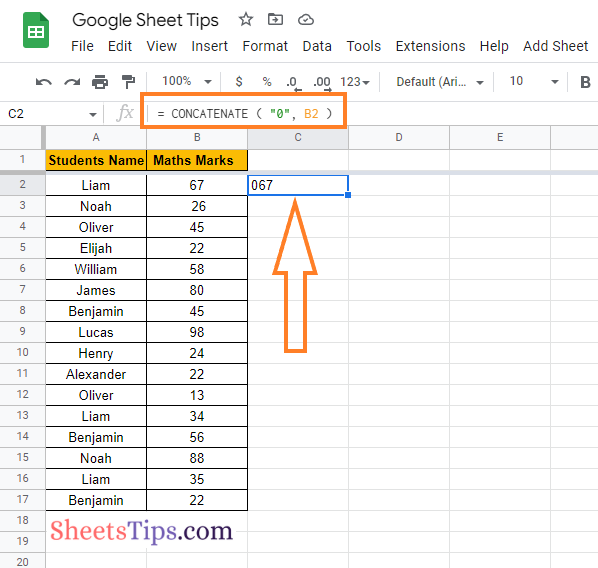One can also simply use the BASE function to add the leading zeros in the Google Spreadsheet. The detailed steps on how to do this are given below:

• 2nd Step: Move to the cell where you want to show the results of adding leading zeros.
• 3rd Step: Enter the formula “= BASE (B2, 10, 3).`"`
• 4th Step: Press the “Enter” key and you will find the results as shown in the image given below.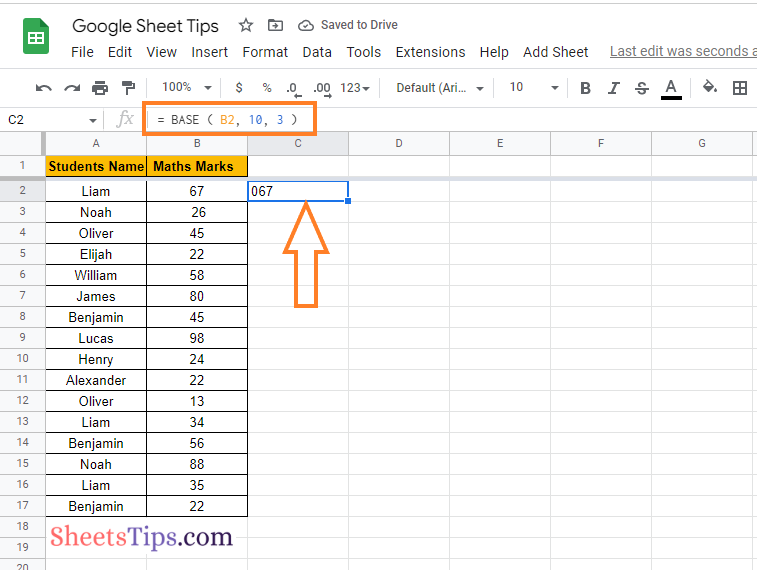We can also simply use the QUERY function in Google Sheets to add the leading zeros to the spreadsheet. The detailed steps are outlined below:

• 2nd Step: On the homepage, click on the cell where you want to use the QUERY function.
• 3rd Step: Enter the formula “`=QUERY (B2:B17, "SELECT * FORMAT B '000'")`“.
• 4th Step: Press the “Return” key and you will find the results as shown in the image given below.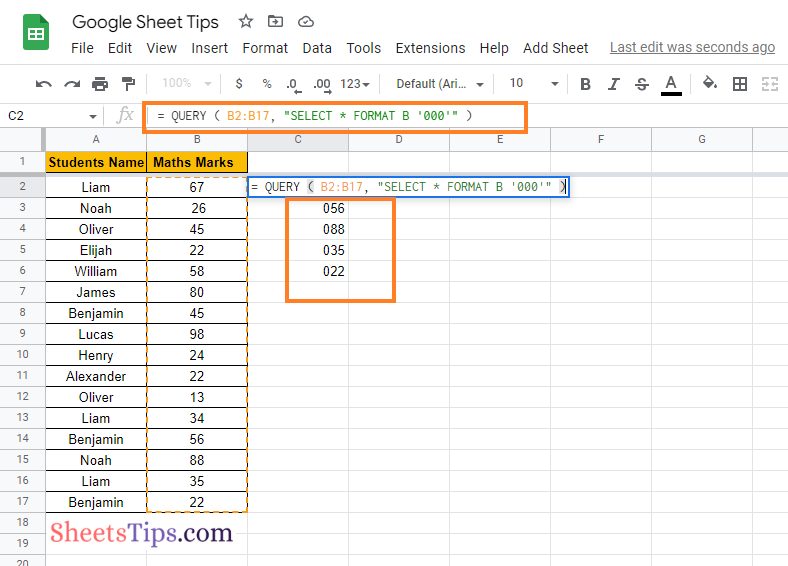• 2nd Step: On the homepage, click on the “Extensions” tab and choose “Apps Scripts” from the drop down menu.
• 3rd Step: Copy and paste the following code into the code editor.
``````function leadingZeros(){